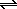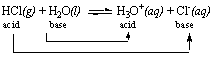×#### Thank you for registering.

One of our academic counsellors will contact you within 1 working day.

Click to Chat

1800-1023-196

+91-120-4616500

CART 0

• 0

MY CART (5)

Use Coupon: CART20 and get 20% off on all online Study Material

ITEM
DETAILS
MRP
DISCOUNT
FINAL PRICE
Total Price: Rs.

There are no items in this cart.
Continue Shopping
```
hello sir, can u send me some information abt acidic strength order of compounds,rules for finding this with some examples plz.

```
11 years ago

```							The Relative Strengths of Conjugate Acid-base Pairs

Strong acids have a weak conjugate base.

Example: HCl is a strong acid. If HCl is a strong acid, it must be a good proton donor. HCl can only be a good proton donor, however, if the Cl- ion is a poor proton acceptor. Thus, the Cl- ion must be a weak base.

HCl(g)
+
H2O(l)H3O+(aq)
+
Cl-(aq)

Strong  acid

Weak base

Strong bases have a weak conjugate acid.

Example: Let's consider the relationship between the strength of the ammonium (NH4+) and its conjugate base, ammonia (NH3). The NH4+ ion is a weak acid because ammonia is a reasonably good base.

NH4+(aq)
+
H2O(l)H3O+(aq)
+
NH3(aq)

Weak acid

Good base

Comparing Relative Strengths of Pairs of Acids and Bases
The value of Ka for an acid can be used to decide whether it is a strong acid or a weak acid, in an absolute sense. It can also be used l to compare the relative strengths of a pair of acids.
Example: Consider HCl and the H3O+ ion.

HCl

Ka = 1 x 106

H3O+

Ka = 55

These Ka values suggest that both are strong acids, but HCl is a stronger acid than the H3O+ ion.
A high proportion of the HCl molecules in an aqueous solution reacts with water to form H3O+ and Cl- ions. The Brnsted theory suggests that every acid-base reaction converts an acid into its conjugate base and a base into its conjugate acid.There are two acids and two bases in this reaction. The stronger acid, however, is on the left side of the equation.

HCl(g)
+
H2O(l)H3O+(aq)
+ Cl-(aq)

stronger acid

weaker acid

The general rules suggest that the stronger of a pair of acids must form the weaker of a pair of conjugate bases. The fact that HCl is a stronger acid than the H3O+ ion implies that the Cl- ion is a weaker base than water.

Acid strength:

HCl > H3O+

Base strength:

Cl- < H2O

Thus, the equation for the reaction between HCl and water can be written as follows.

HCl(g)
+
H2O(l)H3O+(aq)
+
Cl-(aq)

stronger acid

stronger base

weaker acid

weaker base

It isn't surprising that 99.996% of the HCl molecules in a 6 M solution react with water to give H3O+ ions and Cl- ions. The stronger of a pair of acids should react with the stronger of a pair of bases to form a weaker acid and a weaker base.
Let's look at the relative strengths of acetic acid and the H3O+ ion.

CH3CO2H

Ka = 1.8 x 10-5

H3O+

Ka = 55

The values of Ka for these acids suggest that acetic acid is a much weaker acid than the H3O+ ion, which explains why acetic acid is a weak acid in water. Once again, the reaction between the acid and water must convert the acid into its conjugate base and the base into its conjugate acid.But this time, the stronger acid and the stronger base are on the right side of the equation.

CH3CO2H(aq)
+
H2O(l)H3O+(aq)
+
CH3CO2-(aq)

weaker  acid

weaker base

stronger acid

stronger  base

As a result, only a few of the CH3CO2H molecules actually donate an H+ ion to a water molecule to form the H3O+ and CH3CO2- ions.

```
11 years ago
Think You Can Provide A Better Answer ?

## Other Related Questions on Electrostatics

View all Questions »### Course Features

• 101 Video Lectures
• Revision Notes
• Previous Year Papers
• Mind Map
• Study Planner
• NCERT Solutions
• Discussion Forum
• Test paper with Video Solution### Course Features

• 110 Video Lectures
• Revision Notes
• Test paper with Video Solution
• Mind Map
• Study Planner
• NCERT Solutions
• Discussion Forum
• Previous Year Exam Questions